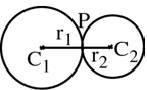# Circles Touching Each Other

Two circles may touch each other either internally or externally. Let C1, C2 be the centers of the circle and r1, r2 be their radii and P the point of contact.

Case 1: The two circles touch externally

The distance between their centres is equal to the sum of their radii.

C1C2 = r1 + r2Case 2: The two circles touch internally

The distance between their centres is equal to the difference of their radii.

C1C2 = C1P − C2P = r1 − r2